Author: Oscar Cronquist Article last updated on September 17, 2019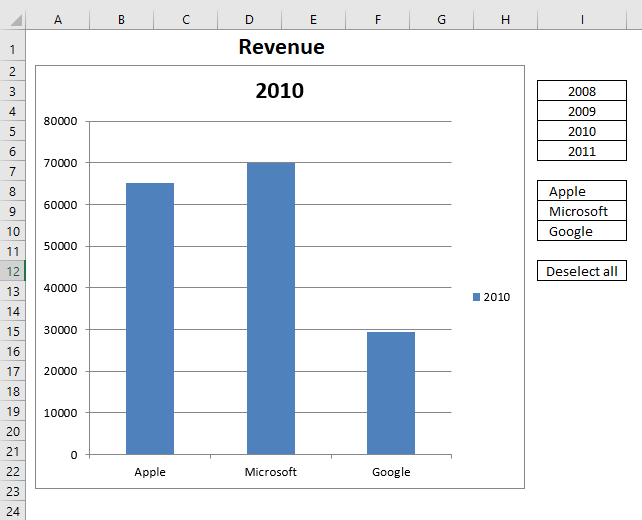I recently discovered an interesting technique about using a user defined function in a HYPERLINK function. Jordan at the Option Explicit VBA blog blogged about this more than a year ago. He made an amazing Periodic Table of Elements.

### How to use the user defined function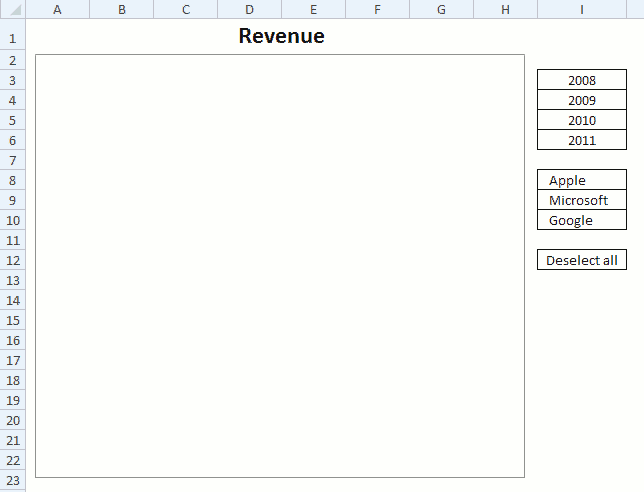I used his technique in this basic chart, see above animated image. I simply move the mouse pointer over a cell and the chart changes instantly, there is really nothing more to it. No need to click on cell to select it or anything like that.

#### Excel defined Table

My data is converted to an Excel defined Table located on a different sheet, shown in the picture below.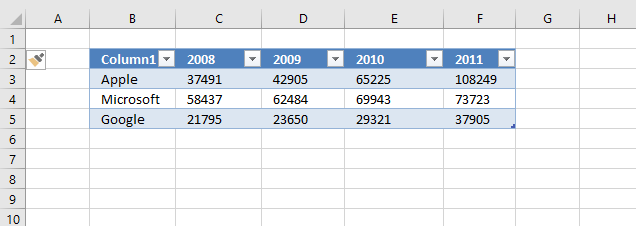The great thing about Excel defined Tables is that they grow automatically if more data is added, you don't have to change cell references in formulas.

1. Select any cell in the data set.
2. Go to tab "Insert".
3. Click on "Table" button.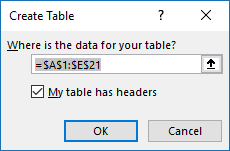4. Click OK button.

#### Hide value in cell I11

The user defined function saves a value in cell I11. I made it invisible by applying custom formatting to it: ;;; The value is still there, however, you can't see it on the worksheet unless you select the cell and check out the formula bar.

1. Select cell I11.
2. Press CTRL + 1 to open the Format Cell dialog box.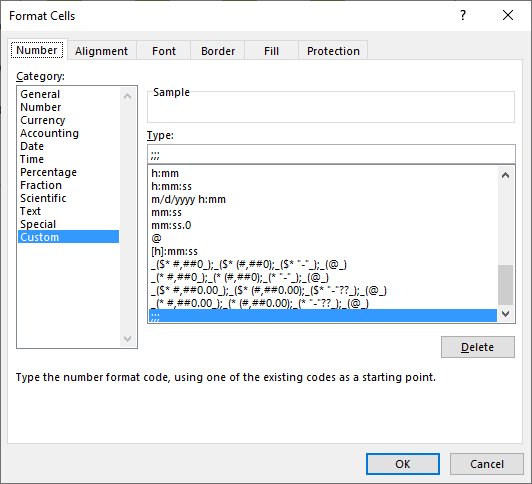3. Make sure you are on tab "Number".
4. Click Category: Custom to select it.
5. Type ;;; (see image above).
6. Click OK button.

#### Formulas

Formula in cell I8:

="Apple"&IFERROR(HYPERLINK(MouseHover("Apple", FALSE),""),"")

#### Explaining formula in cell I8

Step 1 - User defined function

The user defined function MouseHover is triggered when the mouse pointer hovers over the cell. The first argument tells the UDF which value to use in order to sort the Excel defined Table. The second argument tells the UDF to either sort or change chart data source.

MouseHover("Apple", FALSE)

Step 2 - Create Hyperlink

The HYPERLINK function triggers the UDF when the mouse pointer is over a cell.

HYPERLINK(link_location, [friendly_name])

HYPERLINK(MouseHover("Apple", FALSE),"")

Step 3 - Remove error

The IFERROR function allows you to hide errors.

IFERROR(HYPERLINK(MouseHover("Apple", FALSE),""),"")

Step 4 - Remove hyperlink underline

I wanted to remove the hyperlink effect so I added "Apple"& to the formula.

"Apple"&IFERROR(HYPERLINK(MouseHover("Apple", FALSE),""),"")

Formula in cell I3:

="2008"&IFERROR(HYPERLINK(MouseHover("2008", TRUE),""),"")

The user defined function MouseHover filters column 1 if mouse pointer is over any cell in cell range I8:I10. The chart data range is changed if mouse pointer hovers over any cell in cell range I3:I7 based on the value in the given cell.

The bln argument determines what to do, TRUE changes the data source range based on variable str and FALSE filters the Excel defined Table based on variable str.

#### Conditional Formatting

A conditional formatting formula highlights the value currently being hovered over, it simply compares the "invisble" value in cell I11 with the value in eac cell in cell range I8:I10.

=\$I\$11=I8

### VBA code

The boolean value bln determines if

```'Name user defined function and dimension arguments and declare data types
Function MouseHover(str As String, bln As Boolean)

'Check if boolean variable bln is equal to False
If bln = False Then

'Check if variable str is equal to "Deselect all" and cell value in cell I11, sheet2 is not equal to variable str
If str = "Deselect all" And Sheet2.Range("I11") <> str Then

'Save value "Deselect all" to cell I11 on sheet2
Sheet2.Range("I11") = "Deselect all"

'Apply Autofilter to Excel defined table named Table 1
Worksheets("Sheet1").ListObjects("Table1").Range.AutoFilter _
Field:=1, Criteria1:="", Operator:=xlFilterValues

'Stop user defined function
Exit Function
End If

'Check if value in cell I11 (sheet2) is not equal to variable str
If Sheet2.Range("I11") <> str Then

'Save value in variable str to cell I11 (sheet2)
Sheet2.Range("I11") = str

'Change data source to Excel defined table named Table1
ActiveSheet.ChartObjects("Chart 4").Chart.SetSourceData Source:=Sheets("Sheet1").Range("Table1[#All]")

'Plot data by rows on chart 4
ActiveSheet.ChartObjects("Chart 4").Chart.PlotBy = xlRows

'Use value in variable str as a condition in Excel defined Table Table1 and applied to first column
Worksheets("Sheet1").ListObjects("Table1").Range.AutoFilter _
Field:=1, Criteria1:=str, Operator:=xlFilterValues
End If

'Proceed here if boolean variable bln is not equal to False
Else

'Check if value in cell I11 (sheet2) is not equal to variable str
If Sheet2.Range("I11") <> str Then

'Convert value in variable str to string and save it to cell I11 (sheet2)
Sheet2.Range("I11") = CStr(str)

'The asterisk character makes the Excel defined Table show all values
Worksheets("Sheet1").ListObjects("Table1").Range.AutoFilter _
Field:=1, Criteria1:="*", Operator:=xlFilterValues

'Change data source based on first argument
ActiveSheet.ChartObjects("Chart 4").Chart.SetSourceData Source:=Sheets("Sheet1").Range("Table1[[#All],[Column1]], Table1[[#All],[" & str & "]]")

'Change chart settings so it sets the way columns or rows are used as data series on the chart.
ActiveSheet.ChartObjects("Chart 4").Chart.PlotBy = xlColumns
End If
End If
End Function
```

### Where to put the code?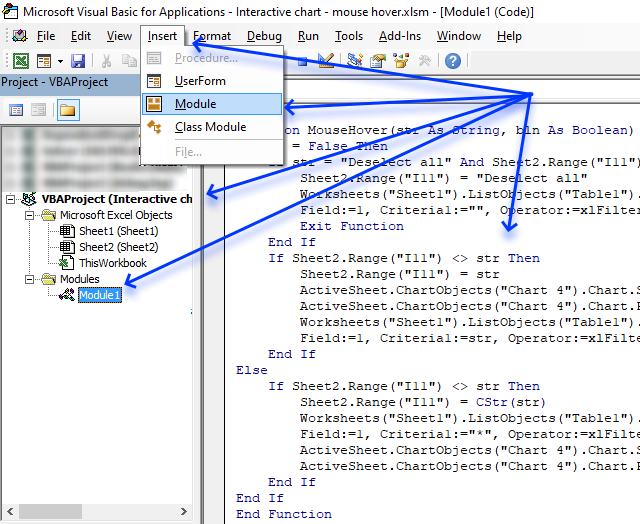1. Create a backup of your workbook.
2. Copy above VBA code.
3. Press Alt + F11 to open the Visual Basic Editor.

### Final notes

I found out that you can't clear a table filter from a user defined function:

```'This won't work!
Worksheets("Sheet1").ListObjects("Table1").Range.AutoFilter Field:=1
```

I had to use "*" to show all values in table column 1.

```'This works!
Worksheets("Sheet1").ListObjects("Table1").Range.AutoFilter _
Field:=1, Criteria1:="*", Operator:=xlFilterValues
```

### Download Excel fileInteractive-chart-mouse-hover.xlsm